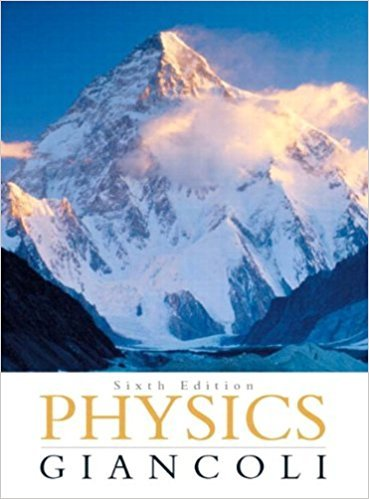×
×

# Answer: At the surface of a certain planet, theISBN: 9780321569837 99

## Solution for problem 29P Chapter 5

Physics: Principles with Applications | 6th Edition

• Textbook Solutions
• 2901 Step-by-step solutions solved by professors and subject experts
• Get 24/7 help from StudySoup virtual teaching assistantsPhysics: Principles with Applications | 6th Edition

4 5 1 329 Reviews
31
2
Problem 29P

At the surface of a certain planet, the gravitational acceleration g has a magnitude of 12.0 m/s2. A 21.0-kg brass ball is transported to this planet. What is (a) the mass of the brass ball on the Earth and on the planet, and (b) the weight of the brass ball on the Earth and on the planet?

Step-by-Step Solution:

Solution 29 P

Step 1 of 3:

In this question, we need to find the mass of the brass ball on the Earth and on planet

In part b, we need to find the weight of the brass ball on Earth and on planet

Data given

Mass of brass ballGravitational acceleration on planetPart a:

Step 2 of 3:

We need to find the mass of the brass ball on the Earth and on planet

Mass of an object is independent of its location

Hence the mass of brass ball remains same on Earth and on planet

That is massPart b:

Step 3 of 3

##### ISBN: 9780321569837

This full solution covers the following key subjects: planet, ball, Brass, EARTH, magnitude. This expansive textbook survival guide covers 33 chapters, and 4141 solutions. The answer to “At the surface of a certain planet, the gravitational acceleration g has a magnitude of 12.0 m/s2. A 21.0-kg brass ball is transported to this planet. What is (a) the mass of the brass ball on the Earth and on the planet, and (b) the weight of the brass ball on the Earth and on the planet?” is broken down into a number of easy to follow steps, and 57 words. Since the solution to 29P from 5 chapter was answered, more than 296 students have viewed the full step-by-step answer. This textbook survival guide was created for the textbook: Physics: Principles with Applications, edition: 6. Physics: Principles with Applications was written by and is associated to the ISBN: 9780321569837. The full step-by-step solution to problem: 29P from chapter: 5 was answered by , our top Physics solution expert on 09/09/17, 04:43AM.

Unlock Textbook Solution